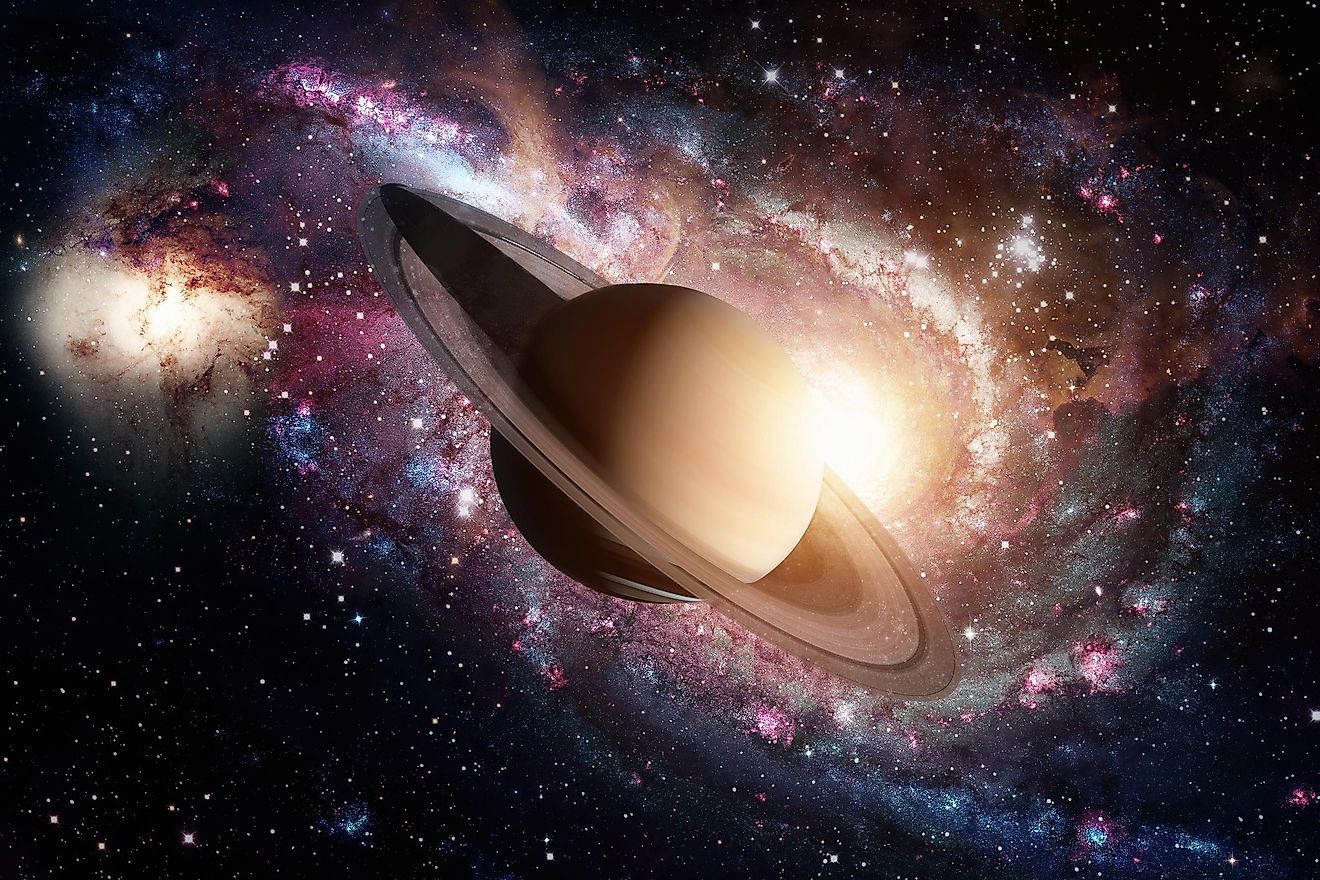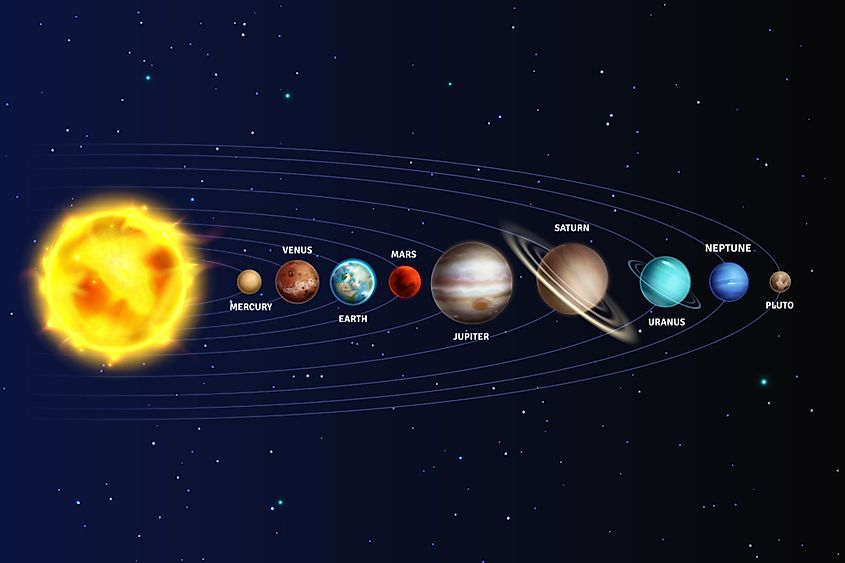# Can Saturn Float In A Bathtub?People often mention that Saturn, the second-largest planet in the Solar System, could float in water. Is this true?
• Saturn is the only one of planets in the Solar System that has a lower density than water.
• The mass of Saturn is about 5.68 x 1026 kg, and its radius is around 5.6 x 107 meters. Its density is 772 kg/m3.
• Whether of not Saturn can float in the bathtub is a purely theoretical question at this point in time.

People often mention that Saturn, the second-largest planet in the Solar System, could float in water. Is this true? If we found a huge bathtub and placed Saturn in it, would it float? Well, while the answer is not exactly simple, the easiest way to answer this is to say - yes. Yes, in theory, Saturn should be able to float in water because it is mostly made of gas, mostly hydrogen and helium.

Gas has a lower density than water, so the entire planet should be able to float in it. However, the planet is not a uniform object; it is not solid like Earth, which means that we can't be sure that it would be able to float in water. This leads us to the answer that it could float in a bathtub in theory, but we can't be sure unless we do some experimentation. Since that experiment is impossible to do, this question will remain a mystery, to an extent.

## The Density Of A Planet

Saturn is one of the most instantly recognizable planets. It has a unique look, and its rings can be seen quite easily with a telescope. All of the other planets in the Solar System have a higher density than water. Since Saturn weighs less than the other planets, it should be able to float in water. It is an interesting theory, although not everyone agrees on it. Still, without a way to test it properly, we can only theorize about it.All of the planets in the Solar System, except for Saturn, have a higher density than water.

If we imagine Saturn as having a spherical shape, we can calculate its density. There are values for the radius and the mass of this planet, so calculating the density should not be a huge issue. The mass of Saturn is about 5.68 x 1026 kg, and its radius is somewhere around 5.6 x 107 meters. By taking these into account, we can easily calculate that the density of Saturn is 772 kg/m3.  Meanwhile, the density of water is 1000 kg/m3, which makes it larger than that of Saturn. This makes it easy to conclude that Saturn would indeed float on water.

## Two Ways To Approach The Question

It all makes sense since the planet has a lower density than water, it should float in it, as do all of the other things with lower density. Gravy, small rocks, and ducks all float because of it. However, we can't use this same logic when we are talking about Saturn. First of all, we do not know how much water we would need for the planet to float. If we assume that we can find a body of water that is larger than a planet with a constant gravitational field, we can try imagining this scenario.

Still, we do not know how deep the water needs to be. The depth also influences the gravitational force, which would, in return, have an impact on the ability to float. Many calculations about this were made, and a lot of them point out that while Saturn would indeed be able to float, only a small part of it would be above water.

The most important thing to note, however, is that Saturn is not a rigid, firm object. This alone would make it hard to imagine it floating. By taking all of this into account, you can easily see how there are multiple ways we can approach this question. And all of the answers will be strictly theoretical because we can't test this. While the density of Saturn is low enough for it to float, its structure would probably make it impossible. Still, we leave it for you to decide what you think is more important, the density, or the structure.

Share